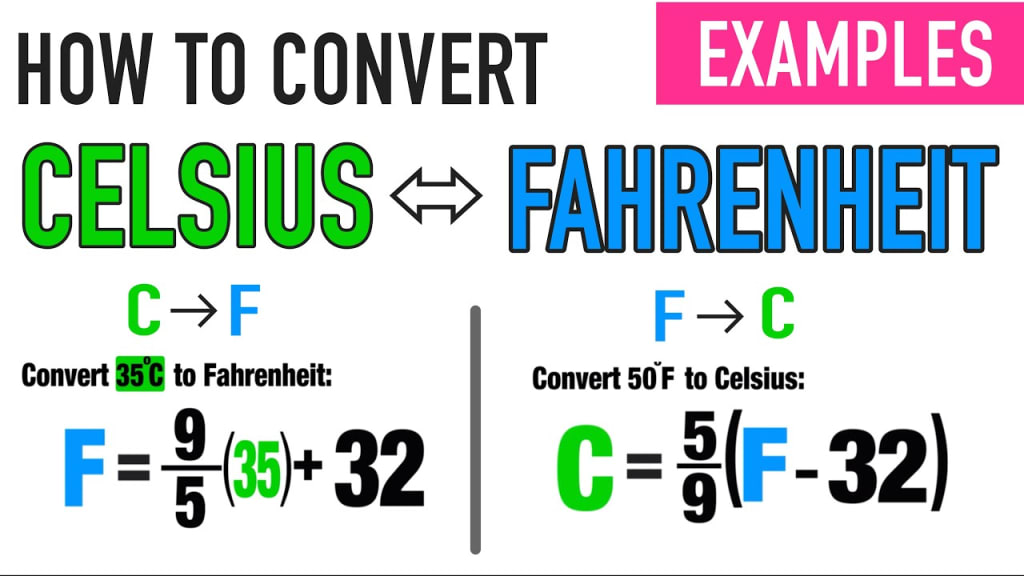# How to convert Celsius to Fahrenheit easy

by Shiraz Kahn 2 years ago in school
Report Story

Do you want to know how to convert Celsius to Fahrenheit easily?Do you want to know how to convert Celsius to Fahrenheit easily? Read the short article about simpler, practical form to do a conversion from Celsius to Fahrenheit. Thanks to the information included in the text below, you will be able to change from Celsius degrees to Fahrenheit degrees quickly.

Generally, the right way to convert Celsius to Fahrenheit is to do two mathematical operations one after another. If you want to know how much is Celsius degrees in Fahrenheit degrees, you have to multiply a temperature specified in Celsius degrees by 1.8 and then add 32 to the obtained result. A conversion should look like this:

Celsius degrees * 1.8 = the result,the result + 32 = Fahrenheit degrees.

It is all you have to do to change temperature in Celsius degrees to temperature in Fahrenheit degrees. But, as we have mentioned in the beginning of this text, there is a simpler solution. In everyday life, very good accuracy is not required, as in science. That’s the reason why you can recalculate Celsius to Fahrenheit with some rounding.

Easy formula for converting Celsius to Fahrenheit

What does the mentioned practical formula look like? The Easy formula for converting Celsius to Fahrenheit looks similar to the one described above, but you have to use other numbers during calculations.

In this case you have to multiply Celsius degrees by 2 and then add 30 to the obtained result. It should look as follows:

Celsius degrees * 2 = the result,the result + 30 = Fahrenheit degrees.

Such a conversion from Celsius to Fahrenheit gives less accurate results, but the whole operation takes also less time, and you probably don't need to use a calculator.

This is a very practical way to change Celsius to Fahrenheit, because you don’t have to multiply Celsius degrees by a number with a decimal point. The multiplication by 2 is a lot faster, because you can calculate it in your head without any calculator help. Adding the number 30 to the result from the previous equation shouldn’t be a problem for you either. Even in the case of difficulties you can be sure that the described way is a bit easier than the previous one.

The fact there are two solutions, one more accurate and one a little easier, is great, because you can always choose by yourself which one is better for you in a specific situation.

The importance of Celsius to Fahrenheit conversion

Generally, it is worth knowing how to convert Celsius to Fahrenheit easily. Practically, this ability is useful in everyday life, because countries in Europe use Celsius degrees to specify a temperature, but, in turn, The United States of America uses Fahrenheit degrees. Without knowledge about converting Celsius to Fahrenheit you can’t read news from the USA correctly.

Besides, you should be able to change Celsius to Fahrenheit, if you are a student, engineer or scientist. Without this ability it is difficult to solve all problems in fields like chemistry, physics or maths. In the mentioned fields the ability to convert temperature from one unit to another is very important.

Do you want to know more about conversion from Celsius degrees to Fahrenheit degrees? Visit our website and read a long article in which we have explained all information about the relationship between Celsius and Fahrenheit. Follow us on the Internet to discover more in the topic of units of temperature.

How to convert Celsius to Fahrenheit easily? How to change Celsius degrees to Fahrenheit degrees simpler and faster? Read the short article to find out how to do it.

Resources

school

## About the author

How does it work?

There are no comments for this story

Be the first to respond and start the conversation.